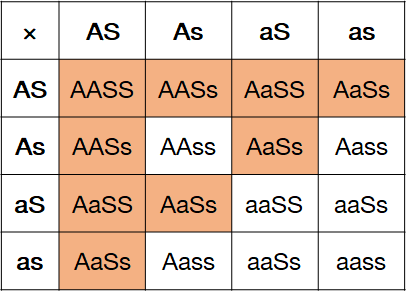# Problem: Sickle cell anemia and albinism are both recessive traits in humans. Imagine that a couple, already pregnant with twins, has just learned that they are both heterozygous for both of these traits.As the couple's genetic counselor, the couple asks you the following questions about how their carrier status will affect their offspring.A. If the couple has fraternal twins, what is the probability that both children will be unaffected by both conditions?B. If the couple has fraternal twins, what is the probability that both of the couple's children will have both sickle cell anemia and albinism?C. What is the probability that one of the fraternal twins is a carrier of either, but not both, of the conditions?(Hint: You will need to use both the product law and the sum law to answer this question.)D. If the couple has fraternal twins, what is the probability of having two phenotypically normal children, one being a carrier of only the sickle cell anemia recessive allele, and the other being a carrier of only the recessive allele for albinism?(Hint: You will need to use both the product law and the sum law to answer this question.)

###### FREE Expert Solution

Sickle Cell Anemia and Albinism: autosomal recessive

Parental Genotypes: AaSs × AaSs (A = albinism, S = sickle cell anemia)

Note: Fraternal twins are developed from two separate fertilization events (probability is independent)

Part A.

Test Cross:Probability that one child is unaffected by either conditions = 9/16

Probability that both child is unaffected by either conditions = 9/16 × 9/16

Answer: The probability that both child is unaffected by both conditions is 81/256 or 0.316.###### Problem Details

Sickle cell anemia and albinism are both recessive traits in humans. Imagine that a couple, already pregnant with twins, has just learned that they are both heterozygous for both of these traits.

As the couple's genetic counselor, the couple asks you the following questions about how their carrier status will affect their offspring.

A. If the couple has fraternal twins, what is the probability that both children will be unaffected by both conditions?

B. If the couple has fraternal twins, what is the probability that both of the couple's children will have both sickle cell anemia and albinism?

C. What is the probability that one of the fraternal twins is a carrier of either, but not both, of the conditions?
(Hint: You will need to use both the product law and the sum law to answer this question.)

D. If the couple has fraternal twins, what is the probability of having two phenotypically normal children, one being a carrier of only the sickle cell anemia recessive allele, and the other being a carrier of only the recessive allele for albinism?
(Hint: You will need to use both the product law and the sum law to answer this question.)